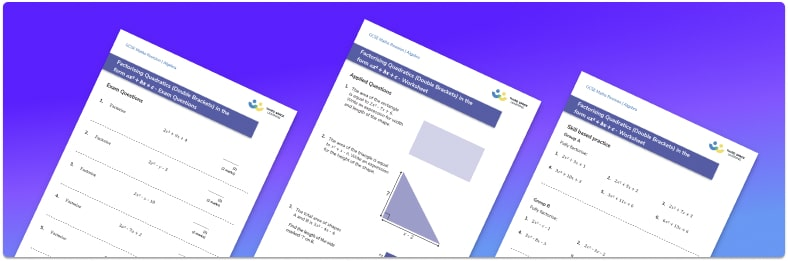• Section 1 contains 20+ skills-based factorising quadratics questions, in 3 groups to support differentiation
• Section 2 contains 4 applied questions with a mix of factorising quadratics worded problems and deeper problem solving questions
• Section 3 contains 3 foundation and higher level GCSE exam practice questions which involve factorising quadratics in different situations
• Answers and a mark scheme for all questions are provided
• Questions follow variation theory with plenty of opportunities for students to work independently at their own level
• All questions are created by fully qualified expert secondary maths teachers
• Suitable for GCSE maths revision for AQA, OCR and Edexcel exam boards

• This field is for validation purposes and should be left unchanged.

You can unsubscribe at any time (each email we send will contain an easy way to unsubscribe). To find out more about how we use your data, see our privacy policy.

### Factorising quadratics at a glance

Factorising quadratics involves factorising quadratic algebraic expressions into double brackets. This means rewriting a quadratic expression so that it is a product of two factors with each factor written as an expression inside a bracket.

Factoring quadratics involves quadratics with a coefficient of 1 for the x² term. This can be done by looking at the constant term and finding its factor pairs and calculating which pair sum to make the coefficient of the x term.

We also need to use factorising quadratic expressions of the form ax²+bx+c. This can be done by using a similar method that first involves multiplying the coefficient of the x² term by the constant.

Factorising quadratic equations can be used to solve quadratic equations. This can help us plot the solutions to quadratic equations on quadratic graphs.

Looking forward, students can progress with more factorising worksheets to additional algebra worksheets, for example a solving equations with fractions worksheet or  simultaneous equations worksheet.For more teaching and learning support on Algebra our GCSE maths lessons provide step by step support for all GCSE maths concepts.

## Do you have KS4 students who need more focused attention to succeed at GCSE?There will be students in your class who require individual attention to help them succeed in their maths GCSEs. In a class of 30, it’s not always easy to provide.

Help your students feel confident with exam-style questions and the strategies they’ll need to answer them correctly with our dedicated GCSE maths revision programme.

Lessons are selected to provide support where each student needs it most, and specially-trained GCSE maths tutors adapt the pitch and pace of each lesson. This ensures a personalised revision programme that raises grades and boosts confidence.

Find out more